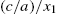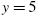﻿ ﻿Errors and Pitfalls - Essentials - Essential MATLAB for Engineers and Scientists (2013)

# Essential MATLAB for Engineers and Scientists (2013)

PART 1

Essentials

### Errors and Pitfalls

Abstract

The objective of this chapter is to begin to learn to recognize and avoid different sorts of errors and pitfalls. Even experienced programmers seldom get programs to run correctly the first time. In computer jargon, an error in a program is called a bug. The process of detecting and correcting such errors is therefore called debugging. There are a number of different types of errors and pitfalls, some of which are peculiar to MATLAB, and some of which may occur when programming in any language. These are discussed briefly in this chapter.

Keywords

Bugs; Debugging; Syntax and logical errors; Run-time errors

CHAPTER OUTLINE

Syntax errors

Incompatible vector sizes

Name hiding

Logic errors

Rounding error

Summary

Chapter exercises

THE OBJECTIVE OF THIS CHAPTER IS TO ENABLE YOU TO:

■ Begin to recognize and avoid different sorts of errors and pitfalls.

Even experienced programmers seldom get programs to run correctly the first time. In computer jargon, an error in a program is called a bug. The story is that a hapless moth short-circuited two thermionic valves in one of the earliest computers. This primeval (charcoaled) ‘bug’ took days to find. The process of detecting and correcting such errors is therefore called debugging. There are a number of different types of errors and pitfalls, some of which are peculiar to MATLAB, and some of which may occur when programming in any language. These are discussed briefly in this chapter.

11.1. Syntax errors

Syntax errors are typing errors in MATLAB statements (e.g., plog instead of plot). They are the most frequent type of error, and are fatal: MATLAB stops execution and displays an error message. As MATLAB evolves from one version to the next, error messages improve. Try the following examples to examine the latest error messages:

2*(1+3

There are many possible syntax errors—you will probably have discovered a few yourself. With experience you will become more adept at spotting your mistakes.

The function lasterr returns the last error message generated.

11.1.1. Incompatible vector sizes

Consider the following statements:

x = 0:pi/20:3*pi;

y = sin(x);

x = 0:pi/40:3*pi;

plot(x,y)

You'll get the error message

Error using ==> plot

Vectors must be the same lengths.

because you forgot to recalculate y after reducing the x increments. whos reveals the problem:

x 1x121 ...

y 1x61 ...

11.1.2. Name hiding

Recall that a workspace variable ‘hides’ a script or function of the same name. The only way to access such a script or function is to clear the offending variable from the workspace.

Furthermore, a MATLAB function hides a script of the same name, e.g., create a script called why.m that displays some junk message, and then type why at the command line.

If you are worried that a variable or script which you are thinking of creating, say blob, may be a MATLAB function, try help blob first.

11.2. Logic errors

These are errors in the actual algorithm you are using to solve a problem, and are the most difficult to find; the program runs, but gives the wrong answers! It's even worse if you don't realize the answers are wrong. The following tips might help you to check out the logic.

■ Try to run the program for some special cases where you know the answers.

■ If you don't know any exact answers, try to use your insight into the problem to check whether the answers seem to be of the right order of magnitude.

■ Try working through the program by hand (or use MATLAB's excellent interactive debugging facilities—see Chapter 7) to see if you can spot where things start going wrong.

11.3. Rounding error

At times, as we have seen, a program will give numerical answers which we know are wrong. This can also be due to rounding error, which results from the finite precision available on the computer, i.e., eight bytes per variable, instead of an infinite number.

Run the following program:

x = 0.1;

while x ~= 0.2

x = x + 0.001;

fprintf( '%g %g\n', x, x - 0.2 )

end

You will find that you need to crash the program to stop it, i.e., with Ctrl-break on a PC. The variable x never has the value 0.2 exactly, because of rounding error. In fact, x misses 0.2 by about, as can be seen from displaying the value of x - 0.2. It would be better to replace the while clause with

while x <= 0.2

or, even better, with

while abs(x - 0.2) > 1e-6

In general, it is always better to test for ‘equality’ of two non-integer expressions as follows:

if abs((a-b)/a) < 1e-6 disp( 'a practically equals b' ),end

or

if abs((a-b)/b) < 1e-6 ...

Note that this equality test is based on the relative difference between a and b, rather than on the absolute difference.

Rounding error may sometimes be reduced by a mathematical re-arrangement of a formula. Recall yet again the common or garden quadratic equationwith solutionsTaking,andgivesand. The second root is expressed as the difference between two nearly equal numbers, and considerable significance is lost. However, as you no doubt remember, the product of the two roots is given by. The second root can therefore be expressed as. Using this form gives, which is more accurate.

Summary

■ Syntax errors are mistakes in the construction of MATLAB statements.

■ Logical errors are errors in the algorithm used to solve a problem.

■ Rounding error occurs because a computer can store numbers only to a finite accuracy.

Chapter exercises

11.1 The Newton quotientmay be used to estimate the first derivativeof a function, if h is ‘small’. Write a program to compute the Newton quotient for the functionat the point(the exact answer is 4) for values of h starting at 1, and decreasing by a factor of 10 each time (use a for loop). The effect of rounding error becomes apparent when h gets ‘too small’, i.e., less than about.

11.2 The solution of the set of simultaneous equations(Exercise 3.6) is given byIfis small, rounding error may cause quite large inaccuracies in the solution. Consider the systemShow that with four-figure floating point arithmetic the solution obtained is,. This level of accuracy may be simulated in the solution of Exercise 3.6 with some statements like

ae = floor( a * e * 1e4 ) / 1e4

and appropriate changes in the coding. The exact solution, obtained without rounding, is,. If the coefficients in the equations are themselves subject to experimental error, the ‘solution’ of this system using limited accuracy is totally meaningless.

﻿